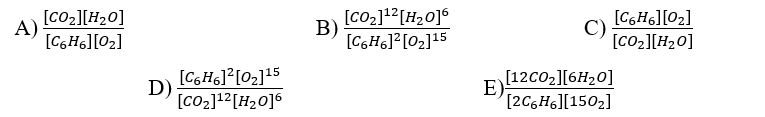🤓 Based on our data, we think this question is relevant for Professor Gill's class at UH.

# Solution: Write the equilibrium expression, Kc, for the following chemical reaction equation.2 C6H6(g) + 15 O2(g) ⇋ 12 CO2(g) + 6 H2O(g)

###### Problem

Write the equilibrium expression, Kc, for the following chemical reaction equation.

2 C6H6(g) + 15 O2(g) ⇋ 12 CO2(g) + 6 H2O(g)